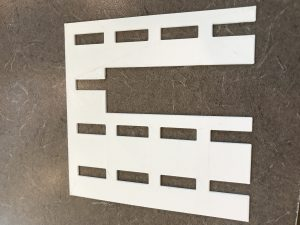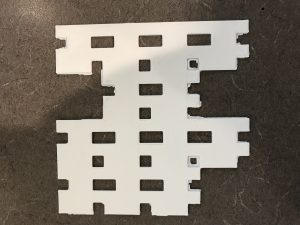Definition

My carpet fractal was created by removing the following coordinates: (2,0), (2,1), and (2,2).

Surface Area

Level 1:In this case, I am simply removing each coordinate that I had removed earlier, which each represent, for the sake of this post, a 1 ft^2 size square. So (2,0), (2,1), and (2,2) amount to 3 ft^2 subtracted, and thus I happen on 13 ft^2.

16 – (3)(1) = 13 ft^2

Level 2:This is where things get interesting. Here, we are going to take the square footage of level 1, and multiply it by 3. We do this because, with each fractal iteration, we are removing 3 big boxes (those 3 coordinates we mentioned earlier). And yet, we are, this time, going to multiply by 1/16, because now, we are moving 3 scaled boxes in 16 subsections of the original fractal. Through these calculations, we got a total area of about 2.4375 ft^2 removed in level 2.

13 x 3 x (1/16) = 2.4375

Then, we subtract the area of level 2 from level 1, and get 11.5625 ft^2.

13 – 2.4375 = 11.5625 ft^2

Level 3:Finally, we are going to do a similar situation, but this time break up those pesky 16 subsections into 16 more subsections, resulting in 256 subsections.

13 x 13 x 3 x (1/256) = 1.9805

And finally, we subtract level 3 from level 2, resulting in 9.5820 ft^2.

11.5025 – 1.9805 = 9.5820 ft^2

Dimension

log4(13) = 1.8502

4^x=13

x=1.8502

Infinite Series